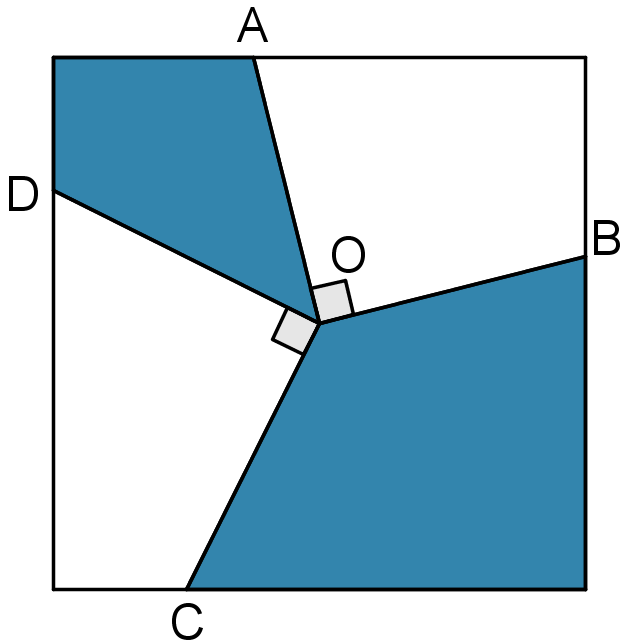You may also likeBuilding Tetrahedra

Can you make a tetrahedron whose faces all have the same perimeter?A 1 metre cube has one face on the ground and one face against a wall. A 4 metre ladder leans against the wall and just touches the cube. How high is the top of the ladder above the ground?Four rods are hinged at their ends to form a convex quadrilateral. Investigate the different shapes that the quadrilateral can take. Be patient this problem may be slow to load.

Age 14 to 16 ShortChallenge LevelThe diagram on the right shows a square with side length $2\text{m}$ and lines drawn to its sides from its centre $O$. The points $A$, $B$, $C$ and $D$ are all on different sides of the square.

The lines $OA$ and $OB$ are perpendicular, as are $OC$ and $OD$.